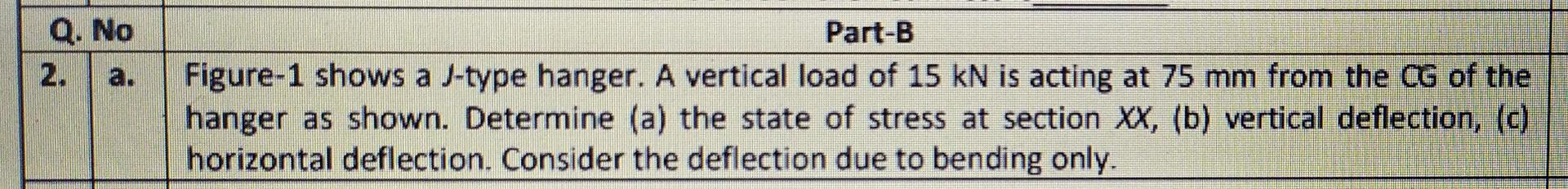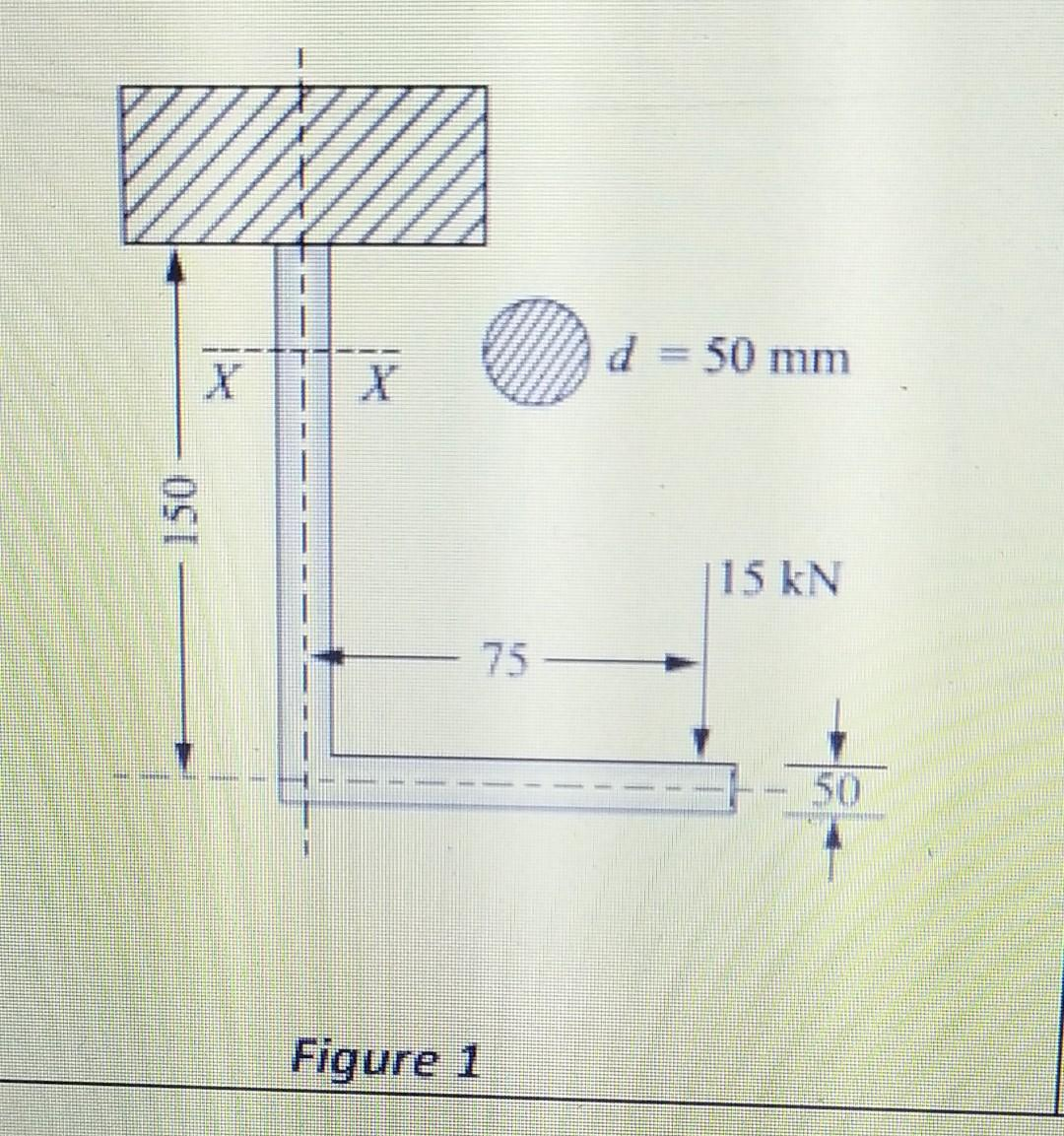# Figure-1 shows a J-type hanger. A vertical load of 15 kN is acting at 75 mm from the CG of the hanger as shown. Determine (a) the state of stress at section XX, (b) vertical deflection, (c) horizontal deflection. Consider the deflection due to bending only.

Question-AnswerCategory: Strength of MaterialsFigure-1 shows a J-type hanger. A vertical load of 15 kN is acting at 75 mm from the CG of the hanger as shown. Determine (a) the state of stress at section XX, (b) vertical deflection, (c) horizontal deflection. Consider the deflection due to bending only.

Figure-1 shows a J-type hanger. A vertical load of 15 kN is acting at 75 mm from the CG of the hanger as shown. Determine (a) the state of stress at section XX, (b) vertical deflection, (c)horizontal deflection. Consider the deflection due to bending only.